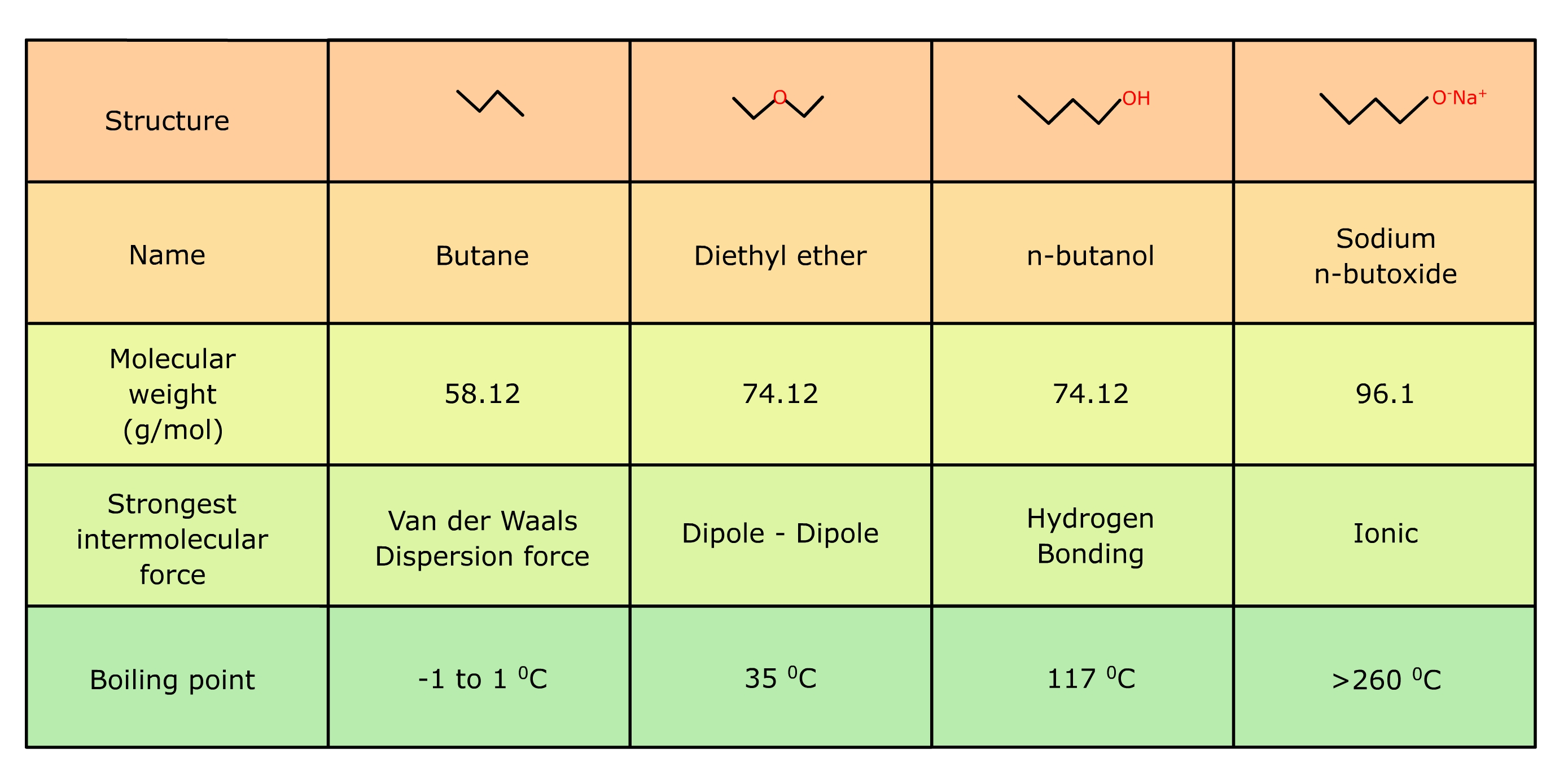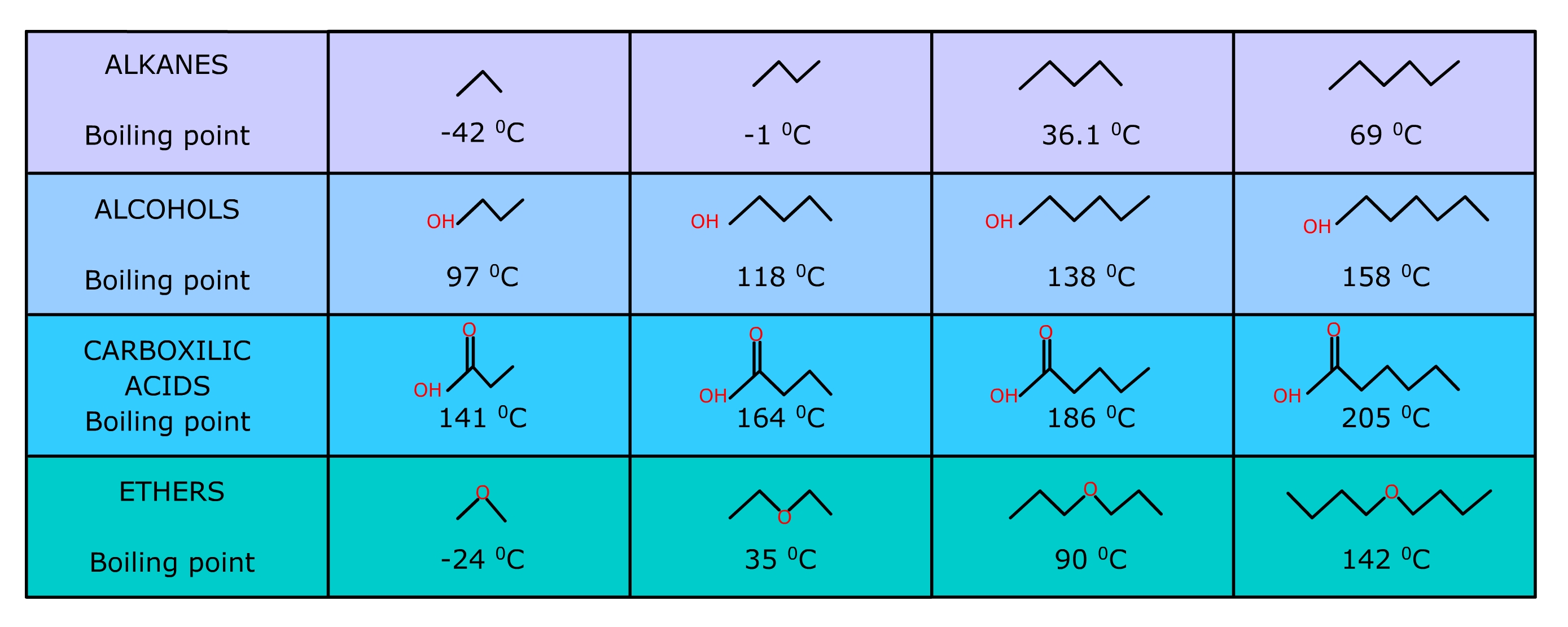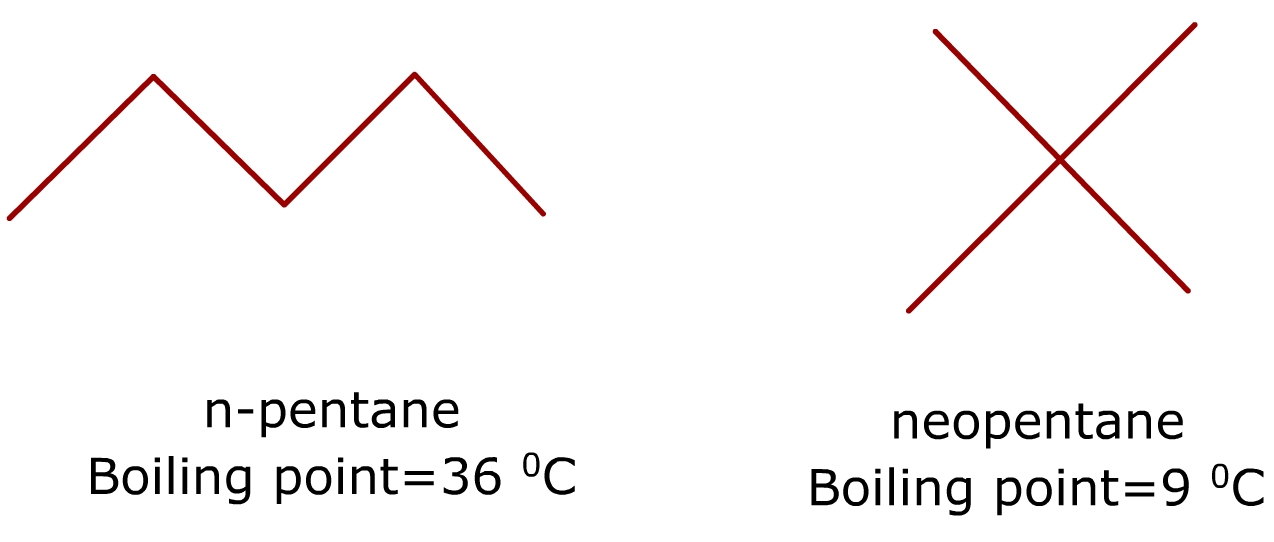Boiling Point of an Organic Compound

# Our Objective

Our objective is to determine the boiling point of an organic compound.

# The Theory

## What is the importance of knowing the boiling point of organic compounds?

The boiling point of organic compounds can give important information about their physical properties and structural characteristics. Boiling point helps identify and characterise a compound. A liquid boils when its vapour pressure is equal to the atmospheric pressure. Vapour pressure is determined by the kinetic energy of a molecule.

Kinetic energy depends on the temperature, mass and velocity of a molecule. When the temperature increases, the average kinetic energy of particles also increases. When the temperature reaches the boiling point, the average kinetic energy becomes sufficient to overcome the force of attraction between the liquid particles. As the force of attraction decreases, the molecules in the liquid state escape from the surface and turn into gas.

The boiling point of a liquid varies with the surrounding atmospheric pressure. A liquid at a higher pressure has a higher boiling point than when that liquid is at lower atmospheric pressure.

The normal boiling point of a compound is an indicator of the volatility of that compound. The higher the boiling point, the less volatile is the compound. Conversely, the lower the boiling point, the more highly volatile is the compound. At a given temperature, if a compound’s normal boiling point is lower, then that compound will generally exist as a gas at atmospheric pressure. If the boiling point of the compound is higher, it then exists as a liquid or a solid.

## What are the general trends that affect the boiling point?

### 1. Strength of intermolecular forces

The relative strength of intermolecular forces such as ionic, hydrogen bonding, dipole-dipole interaction and Vander Waals dispersion force affects the boiling point of a compound. The influence of these forces depends on the functional group present. We can explain the effect of these forces on the boiling point of compounds with the help of some examples.

Consider butane and its three derivatives such as diethyl ether, n- butanol and sodium n- butoxide.n-butane (C4H10) contains no polar functional group. The only attraction between the butane molecules is weak Vander Waals dispersion forces. The result is that butane boils at a temperature at which water freezes, and is much lower than diethyl ether. In the case of diethyl ether, the molecules are held together by dipole-dipole interaction which arises due to the polarized C-O bond. Its boiling point is 35oC.  Compare its boiling point with that of n-butanol. The boiling point of n-butanol is 117oC. The greatly increased boiling point is due to the fact that butanol contains hydroxyl group, which is capable of hydrogen bonding. But the boiling point of sodium butoxide is higher than that of butanol because the attractive force in sodium butoxide is very strong ionic bond.

The intermolecular forces go in the order Ionic > Hydrogen Bonding > Dipole-Dipole > Van der  Waals dispersion force.

### 2. Length of carbon-carbon chain

As the number of carbon atoms increases or the length of carbon-carbon chain increases, the boiling point also increases. This is because the force of attraction between the molecules increases as the molecule gets longer and has more electrons. It takes more energy to overcome the force of attraction, and so the boiling point rises.### 3. Branching decreases the boiling point

As the length of carbon chain increases, the surface area of the compound will also increase. Van der Waals dispersion force is proportional to the surface area. So the increase of surface area increases the ability of individual molecules to attract each other. Branching in molecules decreases the surface area thereby decreasing the attractive force between individual molecules. As a result, the boiling point decreases.

Consider the boiling point of n-pentane and neo-pentane (2,2-dimethyl propane). These are isomers having the same molecular formula (C5H12), but differ in their structures.The boiling point of neopentane is much lower than that of n-pentane.

### 4. Polarity

Polarity of the molecule determines the force of attraction between the molecules in the liquid state. In polar compounds, the positive end of one molecule is attracted by the negative end of another molecule. That means polar molecules are attracted by opposite charge effect. The polarity of a molecule is determined by its functional group. The greater the polarity, the higher is the boiling point.

## Boiling point of some common organic compounds

Compound Boiling Point (oC) Compound Boiling Point (oC)
Benzyl Alcohol 205 Ethyl Benzoate 213
Glycerol 290 Methyl Salicylate 223
Ethylene Glycol 197 Nitrobenzene 211
Phenol 182 Aniline 184
o-Cresol 191 o-Toluidine 200
Benzaldehyde 178 Chlorobenzene 132
Acetophenone 202 Bromobenzene 156
Phenyl Acetate 196 Benzoyl Chloride 197

# Learning Outcomes

1. Students understand the term boiling point from this experiment.
2. Students understand the procedure to determine the boiling point in other organic liquids.
3. Students understand that the boiling point of a liquid is a constant.

Cite this Simulator: